# Character Puzzles Introduction, Verbal Reasoning Notes | Study UPSC CSAT Preparation - UPSC

## UPSC: Character Puzzles Introduction, Verbal Reasoning Notes | Study UPSC CSAT Preparation - UPSC

The document Character Puzzles Introduction, Verbal Reasoning Notes | Study UPSC CSAT Preparation - UPSC is a part of the UPSC Course UPSC CSAT Preparation.
All you need of UPSC at this link: UPSC

Instructions

In this type of questions, a figure or a matrix is given in which some numbers are filled according to a rule. A place is left blank. You have to find out a character (a number or a letter) from the given possible answers which may be filled in the blank space.

Some examples are given below:

Example 1: Which number will replace the question mark ?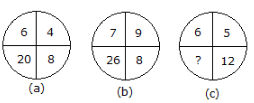Solution:

From fig. a: 6 + 4 + 8 = 18

18 + 2 = 20

From fig. b: 7 + 9 + 8 = 24

24 + 2 = 26

From fig. c: 6 + 5 + 12 = 23

23 + 2 = 25

Hence the number 25 will replace the question mark.

Example 2: Which number will replace the question mark ?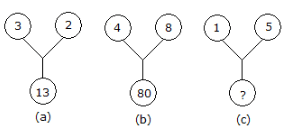Solution:

From fig. a: (3)2 + (2)2 = 13

From fig. b: (4)2 + (8)2 = 80

From fig. c: ? = (1)2 + (5)2

? = 1 + 25

? = 26

Hence the number 26 will replace the question mark.

Example 3: Which number will replace the question mark?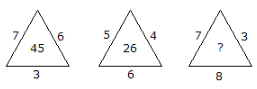Solution:

From fig. a: 7 x 6 + 3 = 45

From fig. b: 5 x 4 + 6 = 26

From fig. c: 7 x 3 + 8 = 29

Hence the number 29 will replace the question mark.

Try yourself:Which number will replace the question mark ?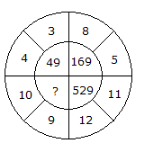Example 4: Which number will replace the question mark ?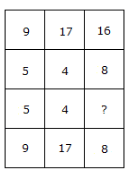Solution:

From column I: (9 x 5) % 5 = 9

From column II: (17 x 4) % 4 = 17

From column III: (16 x ?) % 8 = 8

16? = 64

? = 4

Hence the number 4 will replace the question mark.

Example 5: Which number will replace the question mark ?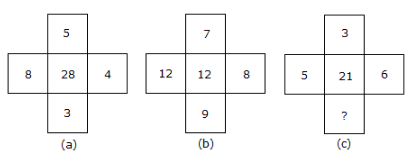Solution:

From fig. a: (8 x 5) - (4 x 3) = 28

From fig. b: (12 x 7) - (8 x 9) = 12

From fig. c: (5 x 3) - (6 x ?) = 21

15 - 6? = 21

6? = -6

? = -1

Hence the number -1 will replace the question mark.

Example 6: Which number will replace the question mark ?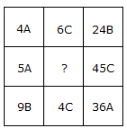Solution:

In each row there are 'A', 'B' and 'C'

In second row 'A' and 'C' are already there

Hence in place of ?, there will be 'B'.

From first row : 4A x 6C = 24B

From third row : 9B x 4C = 36A

From second row : 5A x ? = 45C

? = (45C/5A)

? = 9B

Hence the number 9B will replace the question mark.

Example 7: Which number will replace the question mark?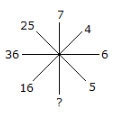Solution:

(5)2 = 25

(6)2 = 36

(4)2 = 16

(7)2 = 49

Hence the number 49 will replace the question mark.

Example 8: Which one will replace the question mark?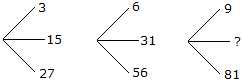Solution:

(15 x 2 - 3) = 27

(31 x 2 - 6) = 56

(45 x 2 - 9) = 81

Try yourself:Example 9: Which one will replace the question mark?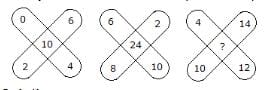The document Character Puzzles Introduction, Verbal Reasoning Notes | Study UPSC CSAT Preparation - UPSC is a part of the UPSC Course UPSC CSAT Preparation.
All you need of UPSC at this link: UPSCUse Code STAYHOME200 and get INR 200 additional OFF

## UPSC CSAT Preparation

58 videos|64 docs|90 tests

### How to Prepare for UPSC

Read our guide to prepare for UPSC which is created by Toppers & the best Teachers

Track your progress, build streaks, highlight & save important lessons and more!

,

,

,

,

,

,

,

,

,

,

,

,

,

,

,

,

,

,

,

,

,

,

,

,

;